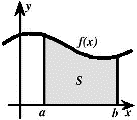## Math in the Middle Institute Partnership## MAT Exam Expository Papers

#### Title

The Exponential Function

#### Date of this Version

5-2006

In partial fulfillment of the requirements for the Masters of Arts in Teaching with a Specialization in the Teaching of Middle Level Mathematics in the Department of Mathematics. Jim Lewis, Advisor May 2006

#### Abstract

One of the basic principles studied in mathematics is the observation of relationships between two connected quantities. A function is this connecting relationship, typically expressed in a formula that describes how one element from the domain is related to exactly one element located in the range (Lial & Miller, 1975). An exponential function is a function with the basic form f (x) = ax , where a (a fixed base that is a real, positive number) is greater than zero and not equal to 1. The exponential function is not to be confused with the polynomial functions, such as x2. One way to recognize the difference between the two functions is by the name of the function. Exponential functions are called so because the variable lies within the exponent of the function (Allendoerfer, Oakley, & Kerr, 1977). These functions are often recognized by the fact that their rate of growth is proportional to their value (Bogley & Robson, 1999). This concept of exponential growth has been around much longer than at the dawn of calculus. Evidence of this dates back almost 4,000 years ago on a Mesopotamian clay tablet, which is now on display at the Louvre. The question translated from the stone slab simply asks, “How long will it take for a sum of money to double if invested at 20 percent interest rate compounded annually?”(Aleff, 2005).

COinS